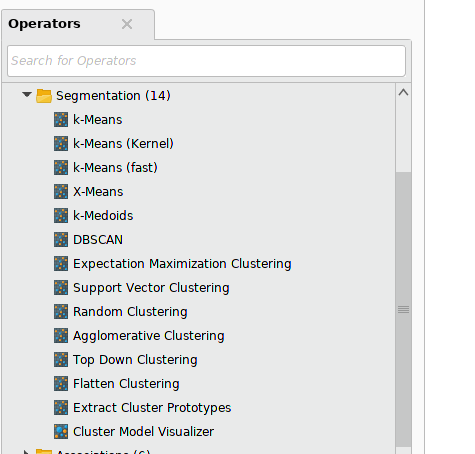# how can ? convert to execute python k-means algorythm to x-means and k-means algorthm ?

Member Posts: 32Contributor I
edited June 2019 in Help
hello everybody , ı have doing k-means clustering via execute python operator but ı want to apply to this process k-medoid and x-means so ı need to change to k-means code section in my python screen so what ı need to write code for x-means and k-medoid to k-means execute python screen .ı have shared my python screen
-------------code----------------
import pandas as pd
from operator import itemgetter
import numpy as np
import random
import sys
from scipy.spatial import distance
from sklearn.cluster import KMeans

C = 10
def k_means(X) :
kmeans = KMeans(n_clusters=C, random_state=0).fit(X)
return kmeans.cluster_centers_

def samevolumecluster( D, volumes):
""" in: point-to-cluster-centre distances D, Npt x C

out: xtoc, X -> C, equal-size clusters

"""

clustervolume = (np.sum(volumes)) / C

xcd = list( np.ndenumerate(D) )  # ((0,0), d00), ((0,1), d01) ...

xcd.sort( key=itemgetter(1) )

xtoc = np.ones( Npt, int ) * -1

nincluster = np.zeros( C, int )

vincluster = np.zeros( C, int)

nall = 0

for (x,c), d in xcd:

if xtoc[x] < 0 and vincluster[c] <= clustervolume+10:

xtoc[x] = c

nincluster[c] += 1

vincluster[c] += volumes[x]

nall += 1

if nall >= Npt:  break

return xtoc

def rm_main(data):

data_2 = data.values
volumes = data_2[:,1]

centres = k_means(data_2)
D = distance.cdist( data_2, centres)
xtoc = samevolumecluster( D, volumes)
data['cluster'] = xtoc
return data
Tagged:

• Administrator, Moderator, Employee, RMResearcher, Member Posts: 246RM Research